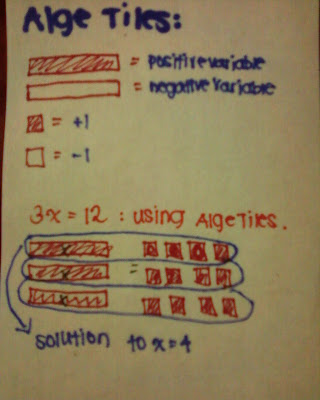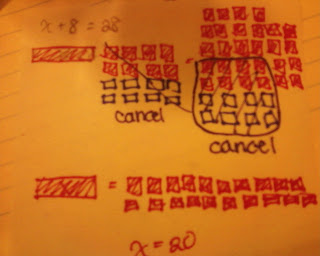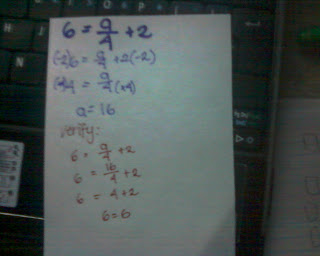## Sunday, May 8, 2011

### Allison's Algebra Post

Terms to remember: Constant: The integer in an expression or equation
Example: a + 4

Variable: The letter that represents an unknown number
Example: a, x, y, k

Equation: Has an equal sign with only one possible answer
Example: 2a= 4

Expression: Is a pattern and has many different answers
Example: 2x

Alge-Tiles:The 3 variables represent 3x.
The 12 tiles represent 12.

To solve one step equations you must isolate the variable you must cancel the constant by using the opposite operation or cancelling it out with a zero pair.
After cancelling the constant you must remember to balance the equation. That means whatever you do to one sideLastly you must verify your answer to see if it is correct. Example:Examples:

x+8=28

x+8(-8)=28(-8)
x= 20
VERIFY:
x+8=28

20+8=28

28=28Subrtraction-

x-2=3

x-2(+2)=3
(+2)
x=5
VERIFY:
x-2=3
5-2=3
3=3Multiplication:

2x=10Division:

k/3= 6Two Step Equations:
1. Isolate the variable
2. Cancel the constant
3. Balance
4. Simplify variable
5. Balance
6. Verify

Examples: